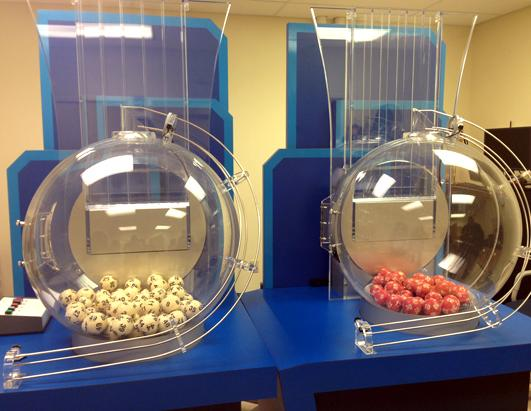# Combination Formula

To calculate the number of possible combinations of r elements from n elements, you can do the following:

1. Calculate (n Permute r)
2. Divide nPr by r!, the number of permutations of r itself. This is because there will be r! permutations for each combination.

The Combination formula can be expressed as one formula. Recall the permutation formula:

n!
(n-r)!

You can then just divide by r! to get the Combination formula:

nCr =
n!

r! * (n-r)!

This formula can be shortened to:
(n choose r) on a calculating device, though again there may be cases where the formula does not apply.### Challenge

In the powerball lottery, 5 balls are chosen from 59 different white balls and 1 ball is chosen from 35 different red balls. The order they are chosen in does not matter. How many outcomes are possible?